# Three colors

Find the probability that 3 balls of the same color will be drawn from fate with 10 white, 10 red, and 10 blue balls.

p =  8.867 %

### Step-by-step explanation: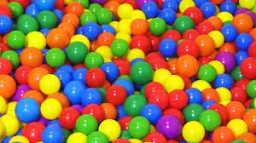Did you find an error or inaccuracy? Feel free to write us. Thank you!Tips to related online calculators
Would you like to compute count of combinations?

## Related math problems and questions:

• White and black ballsThere are 7 white and 3 black balls in an opaque pocket. The balls are the same size. a) Randomly pull out one ball. What is the probability that it will be white? We pull out one ball, see what color it is and return it to the pocket. Then we pull out th
• Round destiny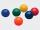There are 5 white and 10 red balls in the destiny. 4 balls will be drawn at random. What is the probability of the event "at least 2 spheres are white"?
• BallsFrom the urn in which are 7 white balls and 17 red, gradually drag 3-times without replacement. What is the probability that pulls balls are in order: red red red?
• Red ballsIn the bag there are 3 red, 12 blue and 8 green balls. How many red balls we must be attached to the bag if we want the probability of pulling out the red balls was 20%?
• The flag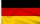The flag should consist of 3 different colored stripes. Available colors: white, red, blue, green and yellow. Specify: A) number of all flags B) number of flags with a blue stripe C) number of flags with a blue stripe in the middle D) the number of flags
• A boxA box contains 6 red balls, 5 blue, and 4 white balls. Find the probability of drawing a) red ball b) non-white ball.
• Components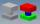In the box are 8 white, 4 blue and 2 red components. What is the probability that we pull one white, one blue and one red component without returning?
• White balls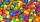I have 3 white balls and 5 red balls in my pocket. How many least balls do I have to remove from the bag to make sure that the pulled out ball is definitely red?
• Three reds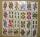What is the probability that when choosing 3 carats from seven carats, all 3 reds will be red?
• Tricolors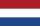From the colors - red, blue, green, black, and white, create all possible tricolors.
• Flags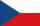How many different flags can be made from colors red, yellow, blue, green, white to consist of three different colors?
• BallsThe urn is 8 white and 6 black balls. We pull 4 randomly balls. What is the probability that among them will be two white?
• Green - Red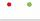We have 5 bags. Each consist one green and 2 red balls. From each we pull just one ball. What is the probability that we doesn't pull any green ball?
• Fall sum or sameFind the probability that if you roll two dice, it will fall the sum of 10, or the same number will fall on both dice.
• Eight blocks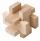Dana had the task to save the eight blocks of these rules: 1. Between two red cubes must be a different color. 2. Between two blue must be two different colors. 3. Between two green must be three different colors. 4. Between two yellow blocks must be four
• One greenIn the container are 45 white and 15 balls. We randomly select 5 balls. What is the probability that it will be a maximum one green?
• Tokens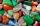In the non-transparent bags are red, white, yellow, blue tokens. We 3times pull one tokens and again returned it, write down all possibilities.